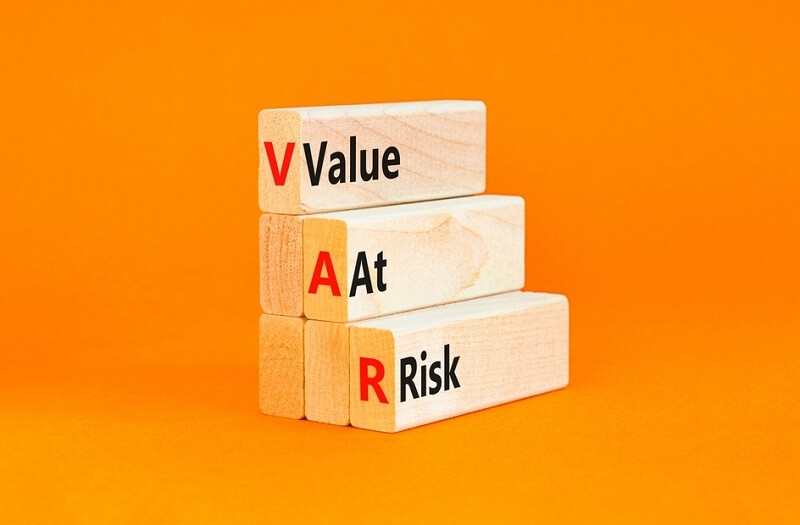# Calculating Value At Risk: A Practical Guide For You

By Yashovardhan SharmaValue at Risk (VaR) is a financial measurement tool used to quantify the amount of risk associated with a particular investment or portfolio. It is often used by banks, insurance companies, and other financial institutions to assess the potential for losses in their portfolios. By understanding and calculating it, investors can make better decisions about their investments and how much risk they are willing to take on. In this blog post, we will explore how to calculate VaR in a practical and straightforward way.

## What is Value at Risk?

Value at Risk is a risk management tool used to measure portfolio performance by the potential losses associated with a particular investment or portfolio of investments. It is typically expressed as a percentage of the total investment for a given period of time, usually a month or a year. By understanding it, investors can make informed decisions about how much risk they are willing to take on. It is a statistical measure that is based on the probability of a portfolio or investment losing a certain amount of value over a given period of time. It is calculated by taking into account the volatility of the investment, the investments expected return, and the time horizon of the investment. VaR is usually expressed as a percentage of the total amount of the investment.

## What Factors Should Be Considered When Calculating VaR?

When calculating Value at Risk, investors should take into account a variety of factors, such as the volatility of the investment, the expected return of the investment, the time horizon of the investment, and the type of investment (e.g., stocks, bonds, commodities, etc.). Additionally, investors should consider any other factors that could affect the potential loss of the investment, such as market conditions, geopolitical events, and interest rate changes. It is important to note that the calculation is not an exact science. There are a variety of methods used to calculate it, and the accuracy of the result depends on the accuracy of the assumptions used in the calculation. As such, it is important to understand the assumptions behind each method in order to ensure that the calculation is as accurate as possible.

## What Are the Different Methods of Calculating VaR?

When calculating Value at Risk, there are a variety of methods that can be used. The most common methods are the Historical Simulation Method, the Parametric Method, and the Monte Carlo Simulation Method. Each of these methods has its own advantages and disadvantages, and the method used should be based on the specific needs of the investor. The Historical Simulation Method is the simplest method of calculating it. This method uses historical data to calculate the probability of an investment losing a certain amount of value over a given period of time. The advantage of this method is that it is easy to understand and use, and the results can be quickly obtained. However, this method is not as accurate as the other methods since it does not take into account any changes in the market or other external factors.

The Parametric Method is a more complex method of calculating it. This method uses assumptions about the investment's volatility, expected return, and time horizon to calculate the probability of an investment losing a certain amount of value. The advantage of this method is that it is more accurate than the Historical Simulation Method, as it takes into account external factors that may affect the investment. However, it is also more difficult to understand and use, and it requires more data and assumptions.

The Monte Carlo Simulation Method is the most sophisticated and accurate method of calculating it. This method uses computer simulations to calculate the probability of an investment losing a certain amount of value over a given period of time. The advantage of this method is that it takes into account a variety of external factors, such as market conditions, geopolitical events, and interest rate changes, which makes it the most accurate of the three methods. However, it is also the most difficult to understand and use, and it requires a large amount of data and assumptions.

## What Are the Benefits of Calculating Value at Risk?

Calculating Value at Risk can provide investors with valuable insights into the potential losses associated with an investment or portfolio of investments. By understanding and calculating it, investors can make better decisions about their investments and how much risk they are willing to take on. Additionally, it can be used to set risk limits, which can help to ensure that investments do not exceed the investors risk appetite. Finally, it can help in portfolio rebalancing for the long term.

## How Is VaR Used in Practice?

Value at Risk is used by banks, insurance companies, and other financial institutions to assess the potential for losses in their portfolios. By understanding and calculating VaR, these institutions can ensure that their investments do not exceed their risk appetite. Additionally, VaR can be used to set risk limits, which can help to mitigate potential losses in the event of an unexpected market event.

## Conclusion

Value at Risk is a powerful tool for quantifying the amount of risk associated with a particular investment or portfolio of investments. By understanding and calculating it, investors can make better decisions about their investments and how much risk they are willing to take on. There are a variety of methods that can be used to calculate VaR, and the method used should be based on the specific needs of the investor. Additionally, VaR can be used in practice by banks, insurance companies, and other financial institutions to assess the potential for losses in their portfolios. It is very useful to build a strong and effective investment portfolio.